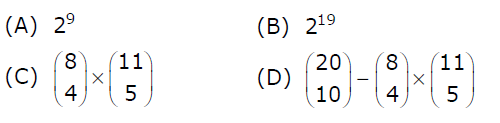# GATE | GATE-CS-2007 | Question 85

Consider the data given in above question.
Suppose that the robot is not allowed to traverse the line segment from (4,4) to (5,4). With this constraint, how many distinct paths are there for the robot to reach (10,10) starting from (0,0)?(A) A
(B) B
(C) C
(D) D

Explanation: Since we are not allowed to traverse from (4,4) to (5,4), we subtract all those paths which were passing through (4,4) to (5,4).
To count number of paths passing through (4,4) to (5,4), we find number of paths from (0,0) to (4,4), and then from (5,4) to (10,10).

```From (0,0) to (4,4), number of paths = 8C4
[found in same way as in previous question].

From (5,4) to (10,10), number of paths = 11C5.
So total number of paths required : 20C10 − 8C4 ∗ 11C5.
```

So option (D) is correct.

My Personal Notes arrow_drop_up
Article Tags :

Be the First to upvote.

Please write to us at contribute@geeksforgeeks.org to report any issue with the above content.